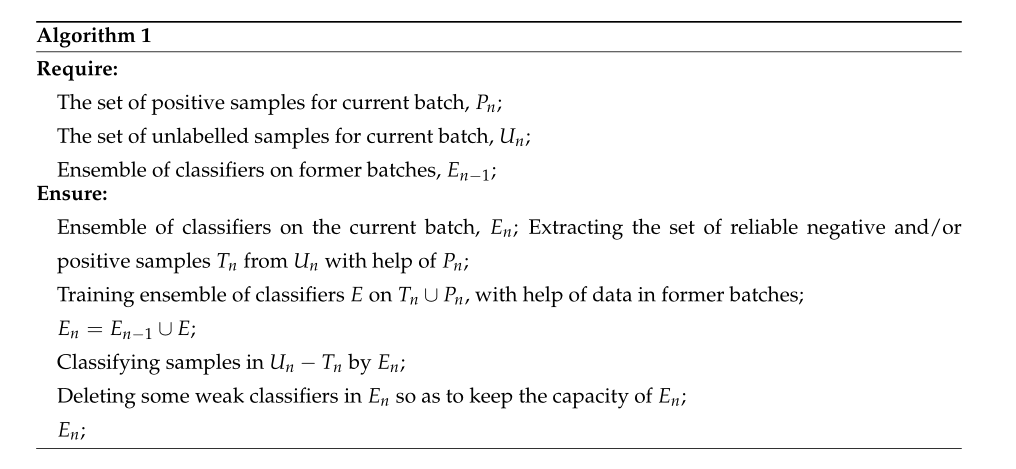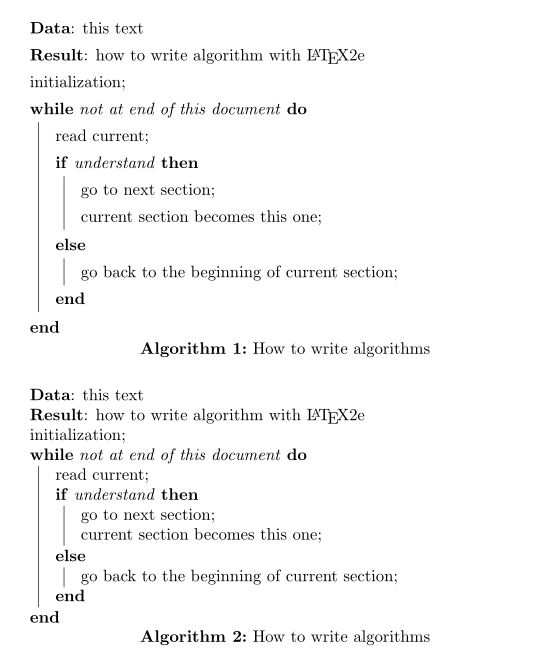# There will be row spacing problems in algorithm use in latex. Please use the ‘setstretch {1.35}

The algorithm template in latex is:
Package added at the top of latex files:

\usepackage{algorithm}
\usepackage{algorithmic}
\usepackage{setspace}

Algorithm block code:\begin{algorithm}[htb]
\setstretch{1.35} %Set the length of the rubber with the specified elasticity (1.35 times the original line width).
\caption{}
\label{alg:Framwork}
\begin{algorithmic}
\REQUIRE ~~\\
The set of positive samples for current batch, $P_n$;\\
The set of unlabelled samples for current batch, $U_n$;\\
Ensemble of classifiers on former batches, $E_{n-1}$;

\ENSURE ~~\\
Ensemble of classifiers on the current batch, $E_n$;
Extracting the set of reliable negative and/or positive samples $T_n$ from $U_n$ with help of $P_n$;\\
\label{ code:fram:extract }

Training ensemble of classifiers $E$ on $T_n \cup P_n$, with help of data in former batches;\\
\label{code:fram:trainbase}

$E_n=E_{n-1}\cup E$;\\

Classifying samples in $U_n-T_n$ by $E_n$;\\
\label{code:fram:classify}

Deleting some weak classifiers in $E_n$ so as to keep the capacity of $E_n$;\\
\label{code:fram:select}

$E_n$;\\
\end{algorithmic}
\end{algorithm}The actual algorithm results are shown as follows:Second usage:
The processing is done using the Algorithm2e package\documentclass{article}
\usepackage{algorithm2e,setspace}

\begin{document}

\begin{algorithm}
\setstretch{1.35}
\SetAlgoLined
\KwData{this text}
\KwResult{how to write algorithm with \LaTeX2e }
initialization\;
\While{not at end of this document}{
\eIf{understand}{
go to next section\;
current section becomes this one\;
}{
go back to the beginning of current section\;
}
}
\caption{How to write algorithms}
\end{algorithm}

\bigskip

\begin{algorithm}
\SetAlgoLined
\KwData{this text}
\KwResult{how to write algorithm with \LaTeX2e }
initialization\;
\While{not at end of this document}{
\eIf{understand}{
go to next section\;
current section becomes this one\;
}{
go back to the beginning of current section\;
}
}
\caption{How to write algorithms}
\end{algorithm}

\end{document}The above codes correspond to two algorithms respectively, and the algorithm blocks obtained are:Common blocks of algorithms in Latex are set after querying, as described above, with other tricks of the process.
The above notes are only used to summarize experience in latex usage.
Latex typesetting needs to be kept calm, otherwise it is easy to make silly mistakes. If you encounter a format or method that you don’t know, use search engines frequently to find the answer.

In fact, I was stuck for a long time by the line spacing problem. The problem I encountered was because the line spacing of the algorithm was too small:
The problem of line spacing can be solved by web search, and also by consulting Liu Haiyang’s latex Latex Primer.